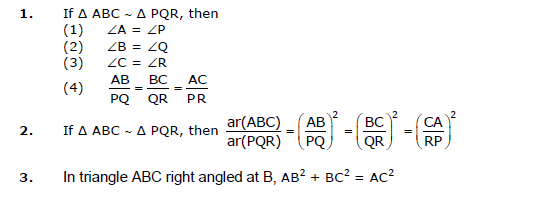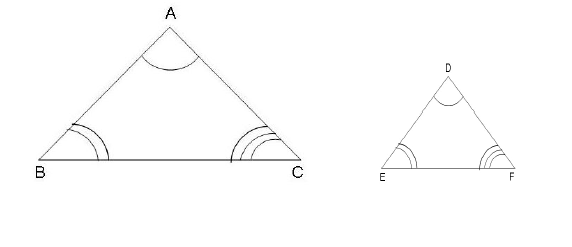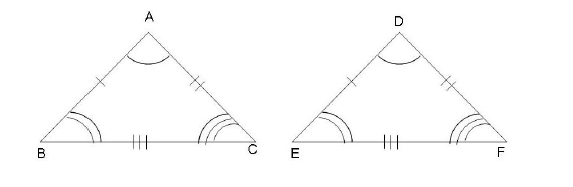# CBSE Class 10 Mathematics Triangles Notes Set A

Download CBSE Class 10 Mathematics Triangles Notes Set A in PDF format. All Revision notes for Class 10 Triangles have been designed as per the latest syllabus and updated chapters given in your textbook for Triangles in Standard 10. Our teachers have designed these concept notes for the benefit of Grade 10 students. You should use these chapter wise notes for revision on daily basis. These study notes can also be used for learning each chapter and its important and difficult topics or revision just before your exams to help you get better scores in upcoming examinations, You can also use Printable notes for Class 10 Triangles for faster revision of difficult topics and get higher rank. After reading these notes also refer to MCQ questions for Class 10 Triangles given our website

## Class 10 Triangles Revision Notes

Class 10 Triangles students should refer to the following concepts and notes for Triangles in standard 10. These exam notes for Grade 10 Triangles will be very useful for upcoming class tests and examinations and help you to score good marks

### Notes Class 10 Triangles

Class-10

Chapter 6: Triangles

Chapter Notes

Top Definitions

1. Two geometrical figures are called congruent if they superpose exactly on each other that is they are of same shape and size.

2. Two figures are similar, if they are of the same shape but of different size.

3. Basic Proportionality Theorem (Thales Theorem): If a line is drawn parallel to one side of a triangle to intersect other two sides in distinct points, the other two sides are divided in the same ratio.

4. Converse of BPT: If a line divides any two sides of a triangle in the same ratio then the line is parallel to the third side.

5. A triangle in which two sides are equal is called an isosceles triangle.

6. AAA (Angle-Angle-Angle) similarity criterion: If in two triangles, corresponding angles are equal, then their corresponding sides are in the same ratio (or proportion) and hence the two triangles are similar.

7. Converse of AAA similarity criterion: If two triangles are similar, then their corresponding angles are equal.

8. SSS (Side- Side- Side) similarity criterion: If in two triangles, sides of one triangle are proportional to (i.e., in the same ratio of) the sides of the other triangle, then their corresponding angles are equal and hence the two triangles are similar.

9. Converse of SSS similarity criterion: If two triangles are similar, then their corresponding sides are in constant proportion.

10. SAS (Side-Angle-Side) similarity criterion: If one angle of a triangle is equal to one angle of the other triangle and the sides including these angles are proportional, then the two triangles are similar.

11. Converse of SAS similarity criterion: If two triangles are similar, then one of the angles of one triangle is equal to the corresponding angle of the other triangle and the sides including these angles are in constant proportion.

12. Pythagoras Theorem: In a right triangle, the square of the hypotenuse is equal to the sum of the squares of the other two sides.

13. Converse of Pythagoras Theorem: If in a triangle, square of one side is equal to the sum of the squares of the other two sides, then the angle opposite the first side is a right angle.

Top Concepts

1. All congruent figures are similar but the similar figures need not be congruent.

2. Two polygons are similar if

* Their corresponding angles are equal

* Their corresponding sides are in same ratio.

3. If the angles in two triangles are:

* Different, the triangles are neither similar nor congruent.

* Same, the triangles are similar.

* Same and the corresponding sides are the same size, the triangles are congruent

4. A line segment drawn through the mid points of one side of a triangle parallel to another side bisects the third side

5. The ratio of any two corresponding sides in two equiangular triangles is always same.

6. All circles are similar.

7. All squares are similar.

8. All equilateral triangles are similar.

9. If two triangles ABC and PQR are similar under the corresponding A ↔ P, B ↔Q and C ↔ R, then symbolically, it is expressed as Δ ABC  Δ PQR.

10. If two angles of a triangle are respectively equal to two angles of another triangle, then by the angle sum property of a triangle their third angles will also be equal.

11. The ratio of the areas of two similar triangles is equal to the square of the ratio of their corresponding sides.

12. The ratio of the areas of two similar triangles is equal to the ratio of the squares of the corresponding medians.

13. Triangles on the same base and between the same parallel lines have equal area.

14. In a rhombus sum of the squares of the sides is equal to the sum of squares of the diagonals.

15. In an equilateral or an isosceles triangle, the altitude divides the base into two equal parts.

16. The altitude of an equilateral triangle with side ‘a’ is √3/2 a.

17. In a square and rhombus, the diagonals bisect each other at right angles

18. If a perpendicular is drawn from the vertex of the right triangle to the hypotenuse then triangles on both sides of the perpendicular are similarto the whole triangle and to each other.

Top FormulaeTop Diagrams

1. Δ ABC ∼ Δ DEF2. Δ ABD ≅ Δ DEF## Tags:

Click for more Triangles Study Material
 CBSE Class 10 Mathematics Triangles Notes Set A CBSE Class 10 Mathematics Triangles Notes Set B

## Latest NCERT & CBSE News

Read the latest news and announcements from NCERT and CBSE below. Important updates relating to your studies which will help you to keep yourself updated with latest happenings in school level education. Keep yourself updated with all latest news and also read articles from teachers which will help you to improve your studies, increase motivation level and promote faster learning

### Pariksha Pe Charcha 2022

The 5th edition of Pariskhas Pe Charcha the unique interactive program of Hon’ble Prime Minister with students teaches and parents will be held through virtual mode in February, 2022. In order to select participants who will be featured in Pariksha Pe Charcha programme...

### Celebration of Matribhasha Diwas Mother Language day

UNESCO has declared 21st February of every year to be celebrated as International Mother Language day to promote dissemination of Mother Language of all, create awareness of linguistic and cultural traditions and diversity across the world and to inspire solidarity...

### Online courses for classes XI and XII offered by NCERT

Ministry of Education (MoE), Government of India has launched a platform for offering Massive Open Online Courses (MOOCs) that is popularly known as SWAYAM (Study Webs of Active learning for Young Aspiring Minds) on 9 th July, 2017. NCERT now offers online courses for...

### Board Exams Date Sheet Class 10 and Class 12

Datesheet for CBSE Board Exams Class 10  (Scroll down for Class 12 Datesheet) Datesheet for CBSE Board Exams Class 12

### Surya Namaskar Project on 75th Anniversary of Independence Day

Ministry of Education, Govt of India vide letter No. F.No. 12-5/2020-IS-4 dated 16.12.2021 has intimated that under the banner Azadi ka Amrit Mahotsav the National Yogasanasports Federation has decided to run a project of 750 million Surya Namaskar from 01 January 2022...

### CBSE Term 2 Board Examinations

CBSE vide Circular No.Acad-51/2021 dated 5th July, 2021, notified that in the session 2021-2022, Board Examinations would be conducted in two terms, i.e.. Term I and Term II. This decision was taken due to the uncertainty arising out of COVID 19 Pandemic. Term I...